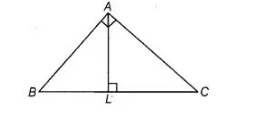# A ΔABC is right angled at A.`
Question:

A ΔABC is right angled at A. L is a point on BC such that AL ⊥ BC. Prove that ∠BAL = ∠ACB.

Solution:

Given In ΔABC, ∠A = 90° and AL ⊥ BC

To prove ∠BAL = ∠ACB

Proof In ΔABC and ΔLAC, ∠BAC = ∠ALC  [each 90°] …(i)

and ∠ABC = ∠ABL [common angle] …(ii)On adding Eqs. (i) and (ii), we get

∠BAC + ∠ABC = ∠ALC + ∠ABL …(iii)

Again, in ΔABC,

∠BAC + ∠ACB + ∠ABC = 180°

[sum of all angles of a triangle is 180°] =>∠BAC+∠ABC = 1 80°-∠ACB …(iv)

In  ΔABL,

∠ABL + ∠ALB + ∠BAL = 180°

[sum of all angles of a triangle is 180°] => ∠ABL+ ∠ALC = 180° – ∠BAL [∴ ∠ALC = ∠ALB= 90°] …(v)

On substituting the value from Eqs. (iv) and (v) in Eq. (iii), we get 180° – ∠ACS = 180° – ∠SAL

=> ∠ACB = ∠BAL

Hence proved.